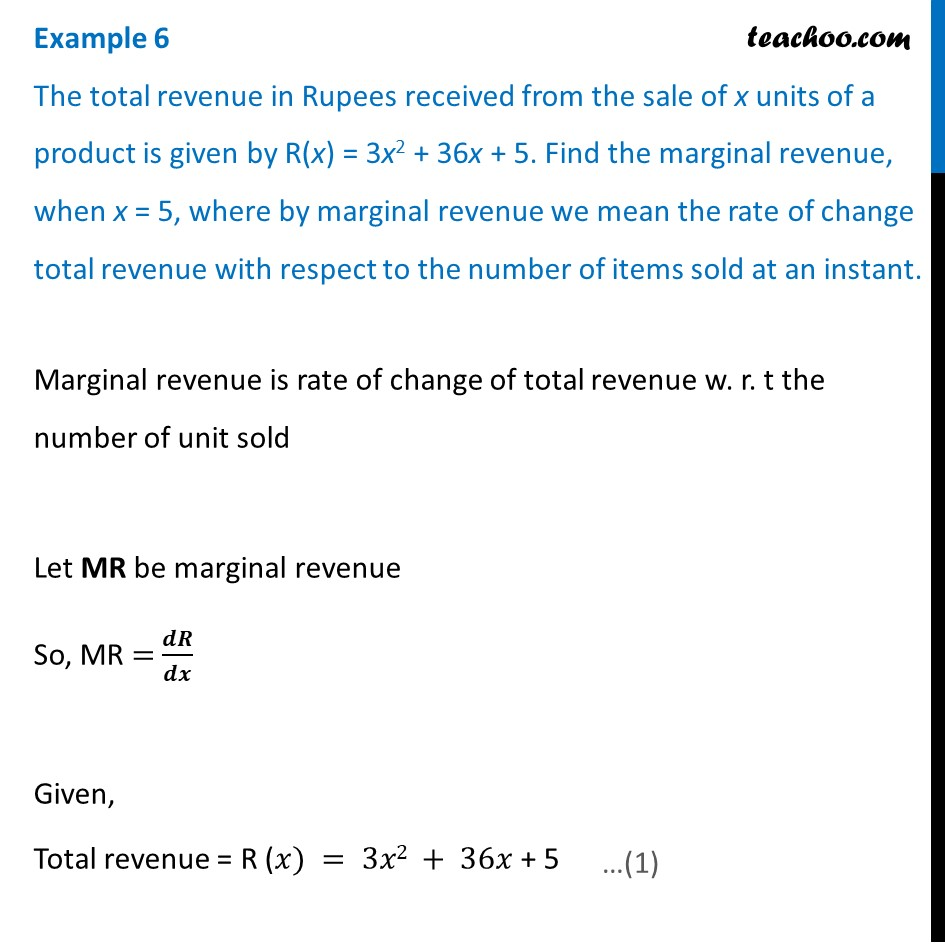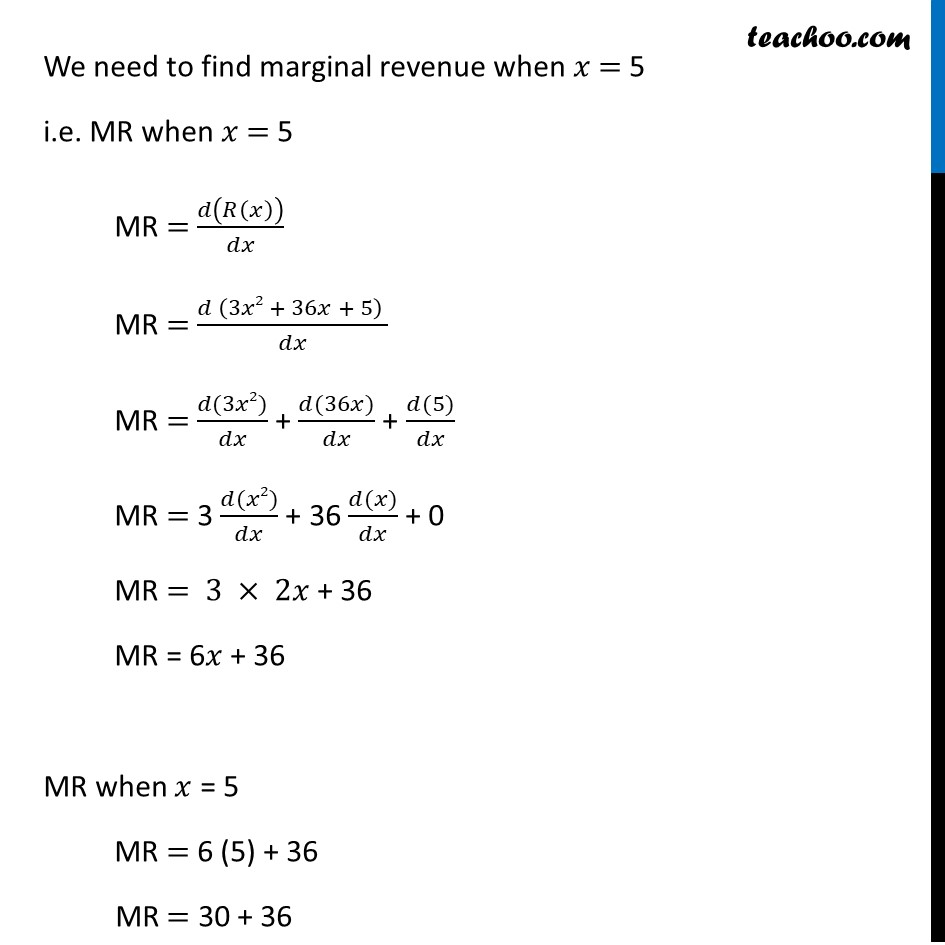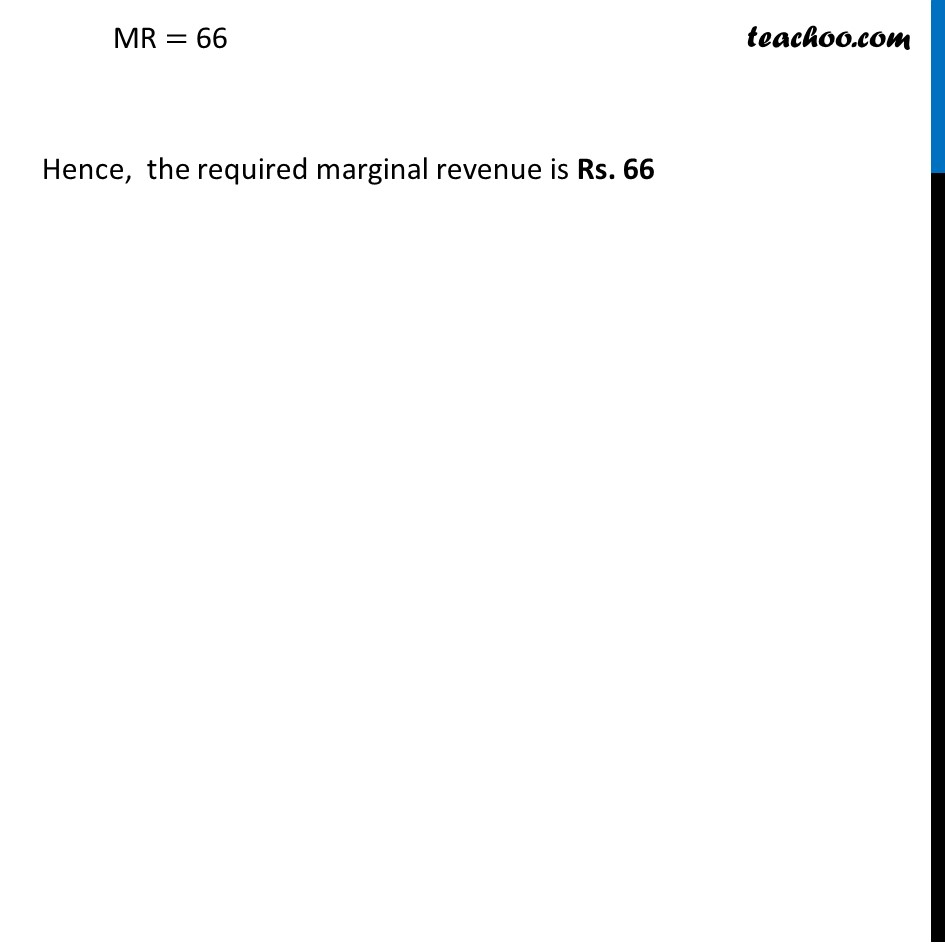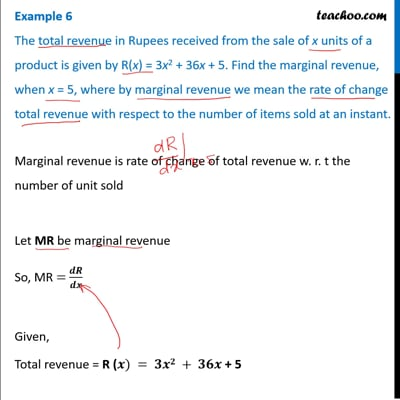Examples

Chapter 6 Class 12 Application of Derivatives
Serial order wiseThis video is only available for Teachoo black users

Maths Crash Course - Live lectures + all videos + Real time Doubt solving!

### Transcript

Example 6 The total revenue in Rupees received from the sale of x units of a product is given by R(x) = 3x2 + 36x + 5. Find the marginal revenue, when x = 5, where by marginal revenue we mean the rate of change total revenue with respect to the number of items sold at an instant.Marginal revenue is rate of change of total revenue w. r. t the number of unit sold Let MR be marginal revenue So, MR = 𝒅𝑹/𝒅𝒙 Given, Total revenue = R (𝑥) = 3𝑥2 + 36𝑥 + 5 We need to find marginal revenue when 𝑥 = 5 i.e. MR when 𝑥 = 5 MR = 𝑑(𝑅(𝑥))/𝑑𝑥 MR = (𝑑 (3𝑥2 + 36𝑥 + 5) )/𝑑𝑥 MR = (𝑑(3𝑥2))/𝑑𝑥 + (𝑑(36𝑥))/𝑑𝑥 + (𝑑(5))/𝑑𝑥 MR = 3 (𝑑(𝑥2))/𝑑𝑥 + 36 (𝑑(𝑥))/𝑑𝑥 + 0 MR = 3 × 2𝑥 + 36 MR = 6𝑥 + 36 MR when 𝑥 = 5 MR = 6 (5) + 36 MR = 30 + 36 MR = 66 Hence, the required marginal revenue is Rs. 66